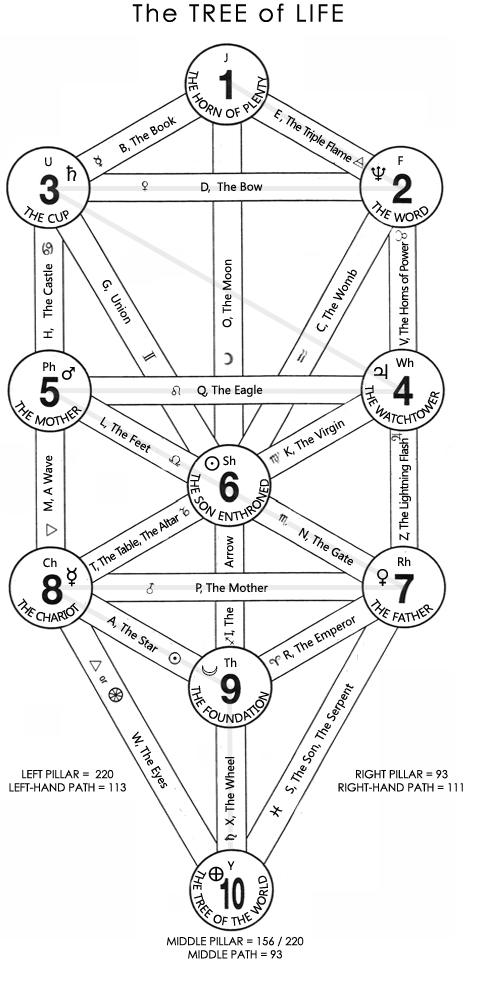# The Tree of the World# Notes:

C's & O's soft.

## Demonstration

(1) The Sum of the Sephiras, that is the Letters of all three Pillars when summed, are equal to the gematria for “The World Tree”

(Left Pillar = 220) + (Right Pillar = 93) + (Middle Pillar = 220) = 533 = ‘The World Tree’

(2) The World Tree conceals / contains the Tree of Life: Sum of the Sephiras,

Middle Path = O + I + X = 93 / 213 / 384 = 'Tree of Life'

(3) The Middle Pillar phases alternate between Victory and establishment of the Law:

Middle Pillar = J + Sh + Th + Y = 5 + 49 + 96 + 6 = 156

Middle Pillar = J + Sh + Th + Y = 5 + 49 + 96 + 70 = 220

(4) The Law is fulfilled in all cases:

Sh (The Son Enthroned) is replaced by ST (The Son on the Cross) when

Y = 6: J + STf + Tfh + Y = 5 + 24 + 89 + 96 + 6 = 220;

(4) The Lightning Flash through the Supernals avows the same:

THE LIGHTNING FLASH through the supernals : J = 1, E = 1, F = 10, D = 8, U = 200 = 220

## Observations

SEPHIRA

• Left Pillar = U + Ch + Ph = 200 + 10 + 10 = 220
• Right Pillar = F + Rh + Wh = 10 + 16 + 67 = 93

(Y - The Tree is 6 on the left; 70 on the right:)

• Middle Pillar = J + Sh + Th + Y = 5 + 49 + 96 + 6 = 156
• Middle Pillar = J + Sh + Th + Y = 5 + 49 + 96 + 70 = 220

PATHS

• Left Path = B + H + M + W = 6 + 7 + 40 + 60 =113
• Right Path = E + V + Z + S = 1 + 80 + 6 + 24 = 111
• Middle Path = O + I + X = 93 / 213 / 384 = 'Tree of Life'

PLANES

• 1st plane : H + G + O + C + V = 7 + 20 + 200 + 3 + 80 = 31 X 10
• 2nd plane : M + L + G + O + C + K + Z = 40 + 50 + 20 + 80 + 3 + 30 + 6 = 229
• 3rd plane : M + T + I + N + Z = 40 + 98 + 4 + 70 + 6 = 228
• 4th plane : W + A + I + R + S = 400 + 4 + 4 + 9 + 24 = 441 = 21 x 21
• 5th plane
• w + x + S = 60 + 9 + 24 = 93
• w + x + Sf = 111
• w + X + S = 60 + 300 + 24 = 384 = ‘tree of life’ = OIX (middle path)

Note :

Planetary Glyphs, pentagrams, and hexagrams and elementary triangles can be derived from the Tree of Life.